## Trinomials of the Form ax^2 + bx + c

Study this pattern for multiplying two binomials: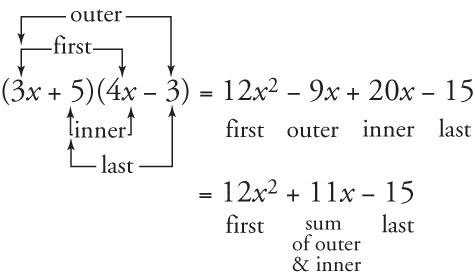##### Example 1

Factor 2 x 2 – 5 x – 12.

Begin by writing two pairs of parentheses.For the first positions, find two factors whose product is 2 x 2. For the last positions, find two factors whose product is –12. Following are the possibilities. The reason for the underlines will be explained shortly. With each possibility, the sum of outer and inner products is included.

1.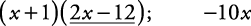2.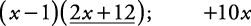3.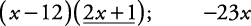4.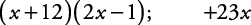5.6.7.8.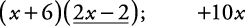9.10.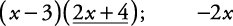11.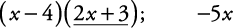12.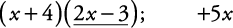Only possibility 11 will multiply out to produce the original polynomial. Therefore,

2 x 2 – 5 x – 12 = ( x – 4)(2 x + 3)

Because many possibilities exist, some shortcuts are advisable:

• Shortcut 1: Be sure the GCF, if there is one, has been factored out.

• Shortcut 2: Try factors closest to one another first. For example, when considering factors of 12, try 3 and 4 before trying 6 and 2 and try 6 and 2 before trying 1 and 12.

• Shortcut 3: Avoid creating binomials that will have a GCF within them. This shortcut eliminates possibilities 1, 2, 5, 6, 7, 8, 9, and 10 (look at the underlined binomials; their terms each have some common factor), leaving only four possibilities to consider. Of the four remaining possibilities, 11 and 12 would be considered first using shortcut 2.

##### Example 2

Factor 8 x 2 – 26 x + 20.

8 x 2 – 26 x + 20 = 2(4 x 2 – 13 x + 10) GCF of 2

For first factors, begin with 2 x and 2 x (closest factors). For last factors, begin with –5 and –2 (closest factors and the product is positive; since the middle term is negative, both factors need to be negative).

(2 x – 5)(2 x – 2)

Shortcut 3 eliminates this possibility.

Now, try –1 and –10 for last factors.

(2 x – 1)(2 x – 10)

Shortcut 3 eliminates this possibility.

Now, try 1 x and 4 x for first factors and go back to –5 and –2 as last factors.

( x – 5)(4 x – 2)

Shortcut 3 eliminates this possibility. But because x and 4 x are different factors, switching the –5 and –2 produces different results, as shown in the following:Therefore, 8 x 2 – 26 x + 20 = 2( x – 2)(4 x – 5).